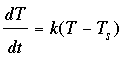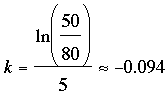SEARCH HOMEMath Central Quandaries & QueriesQuestion from Lori, a parent: If a house is always at 20 degrees celsius. Water (tea) boils at 100 degrees celsius. 5 minutes later the tea was 70 degrees celsius. Use an equation to predict the temperature after 20 minutes.Hello Lori,
To solve this, we will use Newton’s Law of Cooling:Where  ‘T’ is the temperature of the object at time ‘t’, and Ts is the temperature of the surroundings.

(All this statement says is that the change in temperature of the object is proportional to the difference in temperature of the object and its surroundings.)

Using a little calculus we obtain the equation,

T = 80 ekt + 20

We solve for  ‘k’  by noting that the temperature was 70 after 5 minutes.  So,

70 = 80 e5k + 20

Rearranging and taking logarithms we get,Thus, our final equation is given by 70 = 80 e-0.094t + 20.

Plug T = 20 in to find the temperature of the water after 20 minutes.
Write back if you would like a deeper explanation.

TylerMath Central is supported by the University of Regina and The Pacific Institute for the Mathematical Sciences.